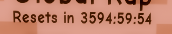# Help me with my countdown timer?

How would I create a countdown timer that includes Days Hours Minutes & Seconds?

Example: a countdown that says, 2 days 5 hours 3 seconds, 2 days 5 hours 2 seconds, 2 days 5 hours 1 second, and so on?

Here’s a countdown script I made but I can’t figure out how to add days to it.

``````function startTimer(totalTime)
totalTime = tonumber(totalTime);
while wait(1) do
totalTime = totalTime - 1;
local Hours = math.floor(totalTime/3600);
local Minutes = math.floor((totalTime/60)%60);
local Seconds = math.floor(totalTime%60);
if string.len(Hours) == 1 then
Hours = "0" .. Hours;
end
if string.len(Minutes) == 1 then
Minutes = "0" .. Minutes;
end
if string.len(Seconds) == 1 then
Seconds = "0" .. Seconds;
end

canvas.Counter.Text = "Resets in " .. Hours .. ":" .. Minutes .. ":" .. Seconds;

if totalTime == 0 then
return nil;
end
end
end
``````

It works just like hours, minutes and seconds did. Do hours as remainder (`totalTime / 3600 % 24`) instead of dividing, and make days `(totalTime / 3600) / 24` (number of hours divided by 24).

2 Likes

I tried this and it messed up the time did thisThen the day was counting down from 28? When the time was set to 86400 here is the code with what you told me:

``````function startTimer(totalTime)
totalTime = tonumber(totalTime);
while wait(1) do
totalTime = totalTime - 1;
local Days = math.floor(totalTime % 3600) % 24;
local Hours = math.floor(totalTime % 3600);
local Minutes = math.floor((totalTime/60)%60);
local Seconds = math.floor(totalTime%60);

print(Days)

if string.len(Hours) == 1 then
Hours = "0" .. Hours;
end
if string.len(Minutes) == 1 then
Minutes = "0" .. Minutes;
end
if string.len(Seconds) == 1 then
Seconds = "0" .. Seconds;
end

canvas.Counter.Text = "Resets in " .. Hours .. ":" .. Minutes .. ":" .. Seconds;

if totalTime == 0 then
return nil;
end
end
end

startTimer(86400);
``````

I’ve updated my post with the corrected code; I mixed up days and hours. Basically you want to divide by 3600 (like you did) to convert to hours, then get the remainder of division by 24 so you can clamp it to what’s just hours and not days. Then for the days, you divide by 3600 and then divide by 24. You can also just divide by 86400 instead of the two different divisions for days.

3 Likes

That fixed the problem! Thank you